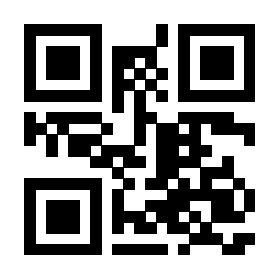# 10l3draw里面如何实现tikz中的\node功能

tikz宏包里的\node命令可以很方便地在指定位置放置元素，比如

\tikz{
\node[rotate=30] (a) at (1,3) {A};
}

0

652

l3draw 相当于 pgf 中最基本的部分。tikz 的作用是把绘图代码转换为 pgf 宏。本问题相当于是说重新实现 tikz

pgf 的一个 node 有形状、锚点、文字，还可以被保存。这些代码是很多的。

\documentclass{article}
\usepackage{l3draw}
\usepackage{tikz}
\begin{document}

\ExplSyntaxOn

\draw_begin:
\draw_path_moveto:n { 0cm , 0cm }
\draw_path_use_clear:n { }

\group_begin:
\draw_transform_rotate:n { 30 }
\draw_transform_shift:n { 1cm , 3cm }
\hbox_set:Nn \l_tmpa_box { A }
\draw_box_use:N \l_tmpa_box
\group_end:

\draw_end:

\ExplSyntaxOff

\tikz{
\path (0,0);
\node[rotate=30,inner sep=0pt,outer sep=0pt,anchor=south west] at (1,3) {A};
}

\end{document}

1 个回答

### 撰写答案